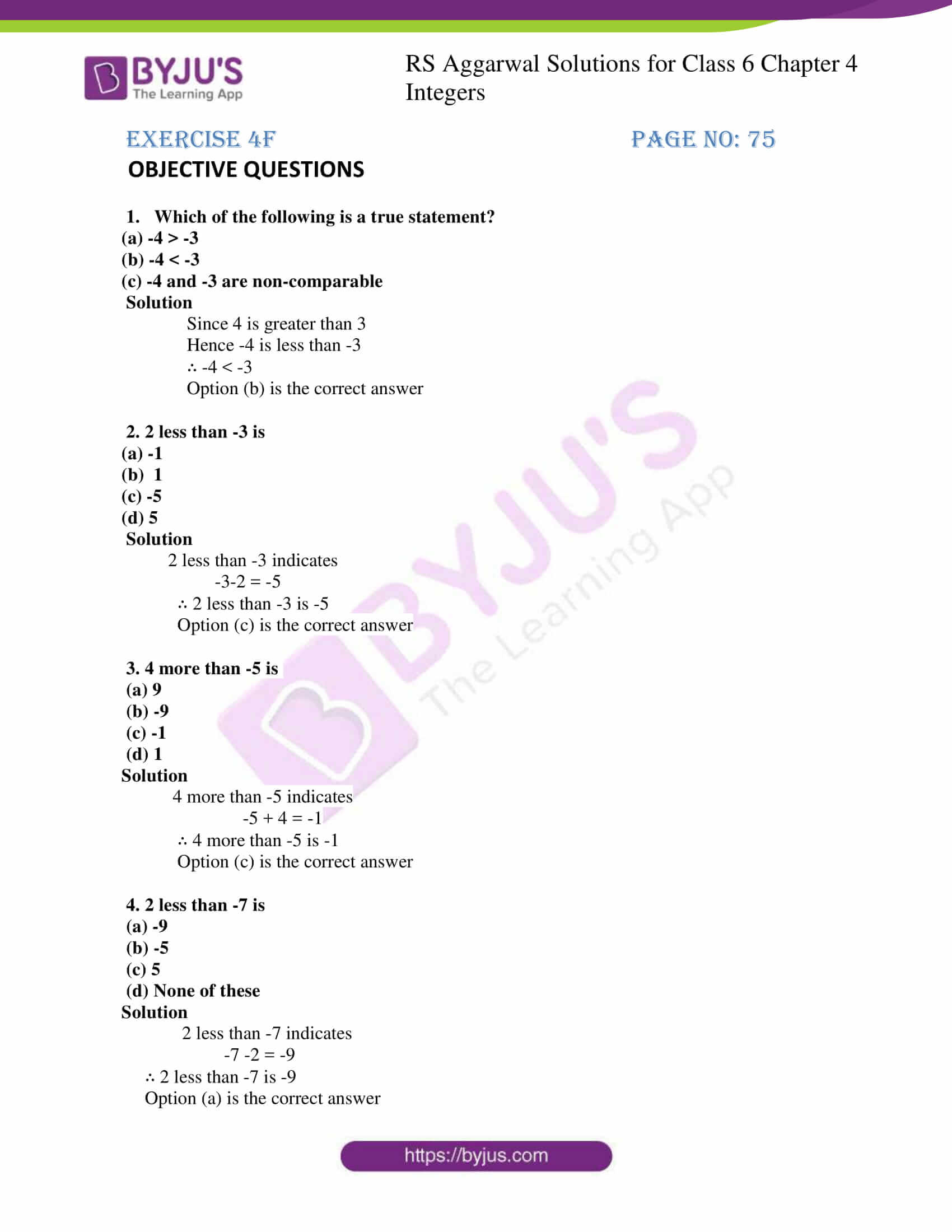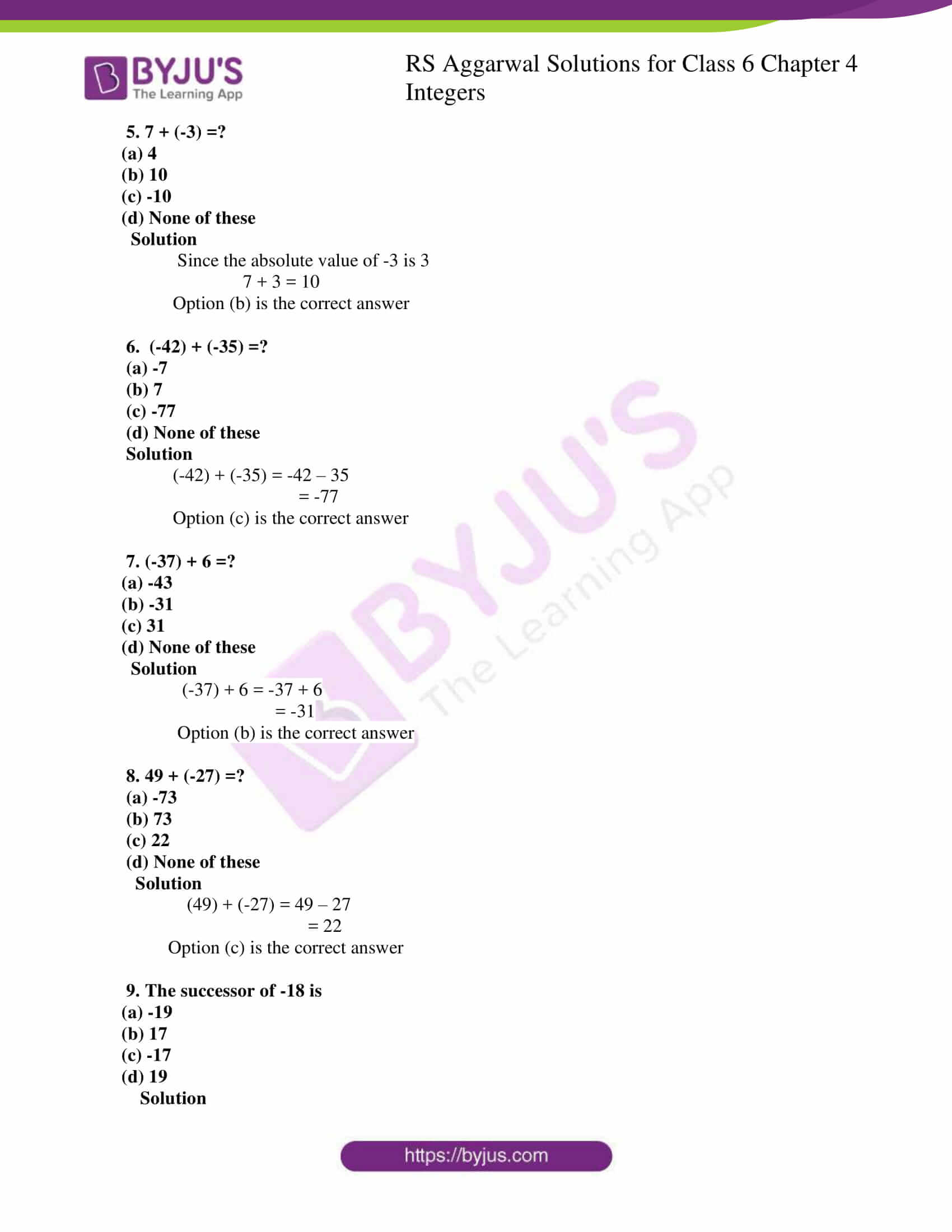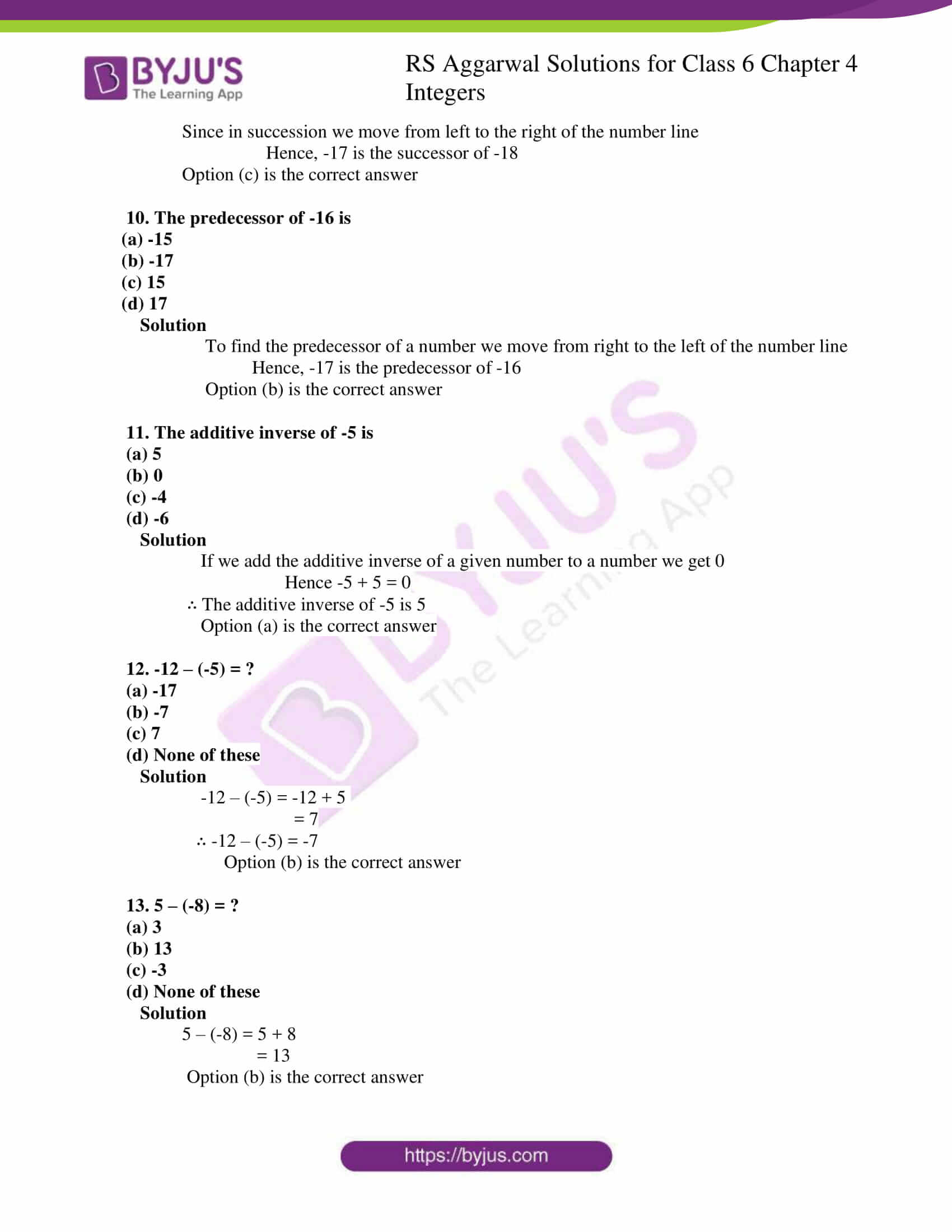# RS Aggarwal Solutions for Class 6 Chapter 4 Integers Exercise 4F

RS Aggarwal Solutions for Class 6, Chapter 4, Integers are here with free download option for students. This exercise has objective type questions which cover the entire chapter. These solutions created by experts are accurate and improves the students with logical thinking abilities. BYJU’S faculty gives a systematic explanation for each solution to help the students in solving them efficiently. Download RS Aggarwal Solutions for Class 6 from their respective links

## Download PDF of RS Aggarwal Solutions for Class 6 Chapter 4 Integers Exercise 4F## Access answers to Maths RS Aggarwal Solutions for Class 6 Chapter 4 Integers Exercise 4F

OBJECTIVE QUESTIONS

1. Which of the following is a true statement?

(a) -4 > -3

(b) -4 < -3

(c) -4 and -3 are non-comparable

Solution

Since 4 is greater than 3

Hence -4 is less than -3

∴ -4 < -3

Option (b) is the correct answer

2. 2 less than -3 is

(a) -1

(b) 1

(c) -5

(d) 5

Solution

2 less than -3 indicates

-3-2 = -5

∴ 2 less than -3 is -5

Option (c) is the correct answer

3. 4 more than -5 is

(a) 9

(b) -9

(c) -1

(d) 1

Solution

4 more than -5 indicates

-5 + 4 = -1

∴ 4 more than -5 is -1

Option (c) is the correct answer

4. 2 less than -7 is

(a) -9

(b) -5

(c) 5

(d) None of these

Solution

2 less than -7 indicates

-7 -2 = -9

∴ 2 less than -7 is -9

Option (a) is the correct answer

5. 7 + (-3) =?

(a) 4

(b) 10

(c) -10

(d) None of these

Solution

Since the absolute value of -3 is 3

7 + 3 = 10

Option (b) is the correct answer

6. (-42) + (-35) =?

(a) -7

(b) 7

(c) -77

(d) None of these

Solution

(-42) + (-35) = -42 – 35

= -77

Option (c) is the correct answer

7. (-37) + 6 =?

(a) -43

(b) -31

(c) 31

(d) None of these

Solution

(-37) + 6 = -37 + 6

= -31

Option (b) is the correct answer

8. 49 + (-27) =?

(a) -73

(b) 73

(c) 22

(d) None of these

Solution

(49) + (-27) = 49 – 27

= 22

Option (c) is the correct answer

9. The successor of -18 is

(a) -19

(b) 17

(c) -17

(d) 19

Solution

Since in succession we move from left to the right of the number line

Hence, -17 is the successor of -18

Option (c) is the correct answer

10. The predecessor of -16 is

(a) -15

(b) -17

(c) 15

(d) 17

Solution

To find the predecessor of a number we move from right to the left of the number line

Hence, -17 is the predecessor of -16

Option (b) is the correct answer

11. The additive inverse of -5 is

(a) 5

(b) 0

(c) -4

(d) -6

Solution

If we add the additive inverse of a given number to a number we get 0

Hence -5 + 5 = 0

∴ The additive inverse of -5 is 5

Option (a) is the correct answer

12. -12 – (-5) = ?

(a) -17

(b) -7

(c) 7

(d) None of these

Solution

-12 – (-5) = -12 + 5

= 7

∴ -12 – (-5) = -7

Option (b) is the correct answer

13. 5 – (-8) = ?

(a) 3

(b) 13

(c) -3

(d) None of these

Solution

5 – (-8) = 5 + 8

= 13

Option (b) is the correct answer